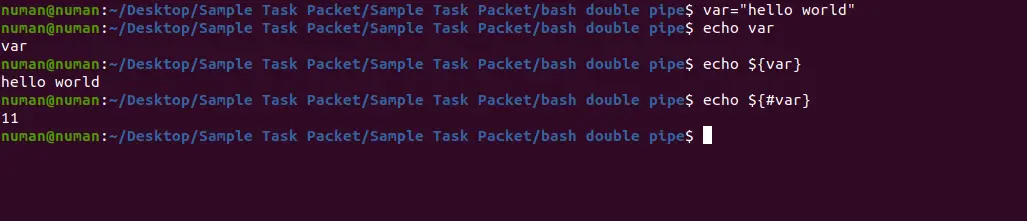# Get Length of String in Bash

Numan Shafi Jan 30, 2023

In programming languages, the length or size of the data type plays an important role. It helps in list traversals and facilitates the extraction of useful information.

The length is particularly important to perform tasks that require traversing the entire string. Therefore, keeping the importance of the length or size of any data type in view, we will learn different ways to calculate the string length.

## String Length and Basic Notations

A string is the sequence of the different `char`, which may include the spaces. In Bash, the length of the string is the total number of chars in that string.

For example, the `"Hello World"` string contains ten `char` and one space. Therefore, its length is eleven.

Most scripting and programming languages have built-in or library functions for finding the length of the string. Similarly, there are many ways to manipulate Bash commands to calculate the string length in Bash.

## Use the `#` Operator to Calculate String Length in Bash

We can use the `#` operator to calculate the length of the string. The following code shows the syntax to get the length of a string using the `#` operator.

``````\${#your_var}
``````

First, we wrapped the variable containing the string with the curly brackets and preceded the `#` operator with the variable.

The `#` operator plays an essential role in printing the length of the string. If `#` is not used, the code will print the whole string, so adding it to the variable is essential.

Moreover, the `\$` sign outside will treat the string length as a variable and print it on the screen using the `echo` command. The following code shows the string’s length calculation using the `#`.

``````var="hello world"
echo \${var}
echo \${#var}
``````In line #1, we create the variable named `var`. The `var` variable can contain any string from the terminal or file string.

The second line prints the whole string using the `\$` and curly brackets. In the last line, we used the `#` symbol with the variable and got the length of the string printed on the command console.

## Use the `expr` Command to Calculate String Length in Bash

Here, we will explore calculating the length of the string using the `expr` command. The following code depicts the basic syntax to use the `expr` command.

```````expr "\$var1" : "\$var2"`
``````

The `expr` command takes two parameters: before and after the comparison operator `:`. The comparison operator compares two strings for common characters and returns the number of similar `chars`.

In `var1`, we give the string whose length needs to be calculated. The `var2` contains a regular expression that parses the string one by one, and the comparison operator can calculate the count of each similar `char`.

The following code demonstrates an example.

``````var="hello world"
echo `expr "\$var" : ".*"`
``````In the above code, we assigned the string `"hello world"` to the variable `var`. The `.*` will parse all characters of the previous token (i.e., the value of `var`).

Therefore, having two same operands, the comparison operator returns the total count of `chars` in the first operand.

## Use the `awk` Command to Calculate String Length in Bash

Let’s calculate the string length using the `awk` command. The `awk` command is a scripting language used for data manipulations and report generation.

The following code demonstrates the string’s length calculation using the `awk` command.

``````var="hello world"
n1=`echo \$var |awk '{print length}'`
echo \$n1
``````

In the above code, we have used the built-in attribute of the `awk` and the `print` command.

The `awk` command takes the input of the string from the `var` variable using the pipe. The pipe sends the command output to the input after the pipe.

Line #3 prints the length of the string as a confirmation.

## Use the `wc` Command to Calculate String Length in Bash

Now we will explore the string’s length calculation using the `wc` command. We only pass the string to the `wc` using the pipe with the flag `-c` or `-m`, and we will get the required output (i.e., length of the string).

The following Bash commands show the string length using the `wc` command.

``````echo -n "\$var" | wc -c
``````

Or:

``````echo -n "\$var" | wc -m
``````

The above code shows the string’s length calculation using two different flags of the `wc` command.

If we use only the `wc` command, it gives more information than the length of the string, which is not required. So, mentioning the flag after the `wc` command is inevitable.

We can use both flags, `-c` or `-m`. Both return the same output.

The following snippet shows the output of the above-described `wc` code.## Calculate Character Length of a File

Now we will explore how to calculate the string length from the file. We will create the file with the name `abc.txt` and write some text.

Then we will read and print the string’s length from the file.

The following code shows the calculation of the string length from the file.

``````touch abc.txt
echo "hello world">> abc.txt
cat abc.txt | wc -c
``````

The `touch` creates a new file, `abc.txt`, to which we write a `hello world` string using simple I/O redirection. The `cat` command on the third line displays the contents of `abc.txt`.

However, the pipe `|` makes the output of the `cat` command input to the `wc` command. Therefore, the `wc` will count words in this output.